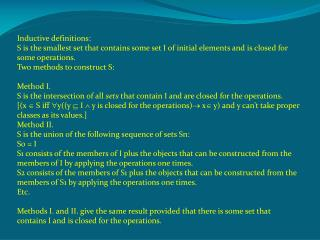DownloadDownload PresentationInductive definitions:

# Inductive definitions:

Download Presentation## Inductive definitions:

- - - - - - - - - - - - - - - - - - - - - - - - - - - E N D - - - - - - - - - - - - - - - - - - - - - - - - - - -
##### Presentation Transcript

1. Inductive definitions: S is the smallest set that contains some set I of initial elements and is closed for some operations. Two methods to construct S: Method I. S is the intersection of all setsthat contain I and are closed for the operations.[(x  S iff y((y  I  y is closed for the operations) x y) and y can’t take proper classes as its values.] Method II. S is the union of the following sequence of sets Sn: S0 = I S1 consists of the members of I plus the objects that can be constructed from the members of I by applying the operations one times. S2 consists of the members of S1 plus the objects that can be constructed from the members of S1 by applying the operations one times. Etc. Methods I. and II. give the same result provided that there is some set that contains I and is closed for the operations.

2. Examples: I consists of the emptyset only and the operation is the follower (+): a+= a  {a} Method II. gives  as the inductively defined set (?). If we leave off the axiom of infinity, then every infinite class will be a proper class and the class given by Method I. will be the class of all objects (even if we have atoms) , because the condition for being a member of S will be vacuously true for all objects. Method II. gives  evenin this case (although it will be a proper class and not a set).Syntax of propositional logic I is a set of atomic propositions, the operations are the propositional connectives. There is a closed set (in the usual set theory) because the class of finite sequences of atomic propositions, connectives and parentheses is a countable class and therefore a set.

3. Coinductive definitions: S’ is the largest set each member of thatis either a member of I or can be produced by the operations from some other members. In regular set theory, S’ is usually (?) the same as S. Let ’ be the set defined coinductively by  as the single initial element and the follower-operation.  is a set which satisfies the coinductive conditions, therefore   ’. Let n be a member of ’. If n= , then n  . If n is a follower of some m, then m  n. If m= , then n= {} and n  . If m is a follower of l, then l m, … By regularity, this descending -chain ends after finitely many step and the result is that n   again. If we reject regularity, then   ’.

4. A very important example: hereditary finite sets with a finite set of atoms A. A set is hereditary finite if is finite, all of its set members are finite, all the members of its set members are finite,… (in the wellfounded case: down to the atoms or to the emptyset.) Inductive definition, method I.: HF0 is the smallest set containing the atoms and containing as a member each of its finite subsets. HF0 is the intersection of the sets containing the atoms and closed for the operation finite collection. Method II.: H0= A, H1= A  pot(A), H(n+1) = Hn  pot (Hn), HF0 is the union of all Hn-s. HF0 is wellfounded. Coinductive definition: HF1 is the largest set each member of which is either an atom or a finite collection of other members. HF0 is a subset of HF1. HF0 is a proper subset of HF1.